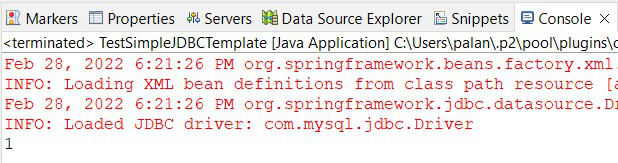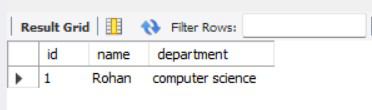Open in App
Not now

# Spring – SimpleJDBCTemplate with Example

• Last Updated : 07 Mar, 2022

The SimpleJDBCTemplate includes all the features and functionalities of JdbcTemplate class and it also supports the Java5 features such as var-args(variable arguments) and autoboxing. Along with JdbcTemplate class, it also provides the update() method which takes two arguments the SQL query and arbitrary arguments which depend upon the SQL query. In order to access the methods of the old JdbcTemplate class, we use the getJdbcOperations() method and we call all those methods over SimpleJdbcTemplate.

Note: We need to pass the parameters inside the update() method in the same order we defined them in parameterized query.

Syntax for update() method of SimpleJDBCTemplate class:

`int update(String sqlQuery, Object parameters)`

### Example

In this example, we will update a student’s details using the update() method of SimpleJDBCTemplate class. For this tutorial, we will be using the following schema for the Student table.

`Student(id INT, name VARCHAR(45), department VARCHAR(45))`

Step By Step Implementation

Step 1: Create Table

In this step, we will create a Student table to store students’ information. For this tutorial, we will assume you have created the following table in your database.

```CREATE TABLE STUDENT(
id INT,
name VARCHAR(45),
department VARCHAR(45));```

After creating the table we will insert the following data in our table.

`INSERT INTO STUDENT VALUES(1, "geek", "computer science");`

In this step, we will add the maven dependencies to our application. Add the following dependencies to your pom.xml

Note: Spring 3 JDBC supports SimpleJDBCTemplate class and Java5 features

## XML

 `<``project` `xmlns``=``"http://maven.apache.org/POM/4.0.0"` `         ``xmlns:xsi``=``"http://www.w3.org/2001/XMLSchema-instance"`  `         ``xsi:schemaLocation="http://maven.apache.org/POM/4.0.0  ` `                             ``https://maven.apache.org/xsd/maven-4.0.0.xsd"> ` `  ``<``modelVersion``>4.0.0 ` `  ``<``groupId``>com.geeksforgeeks ` `  ``<``artifactId``>SimpleJDBCTemplate ` `  ``<``version``>0.0.1-SNAPSHOT ` `   `  `  ``<``dependencies``> ` `     `  `        ``` `        ``<``dependency``> ` `            ``<``groupId``>org.springframework ` `            ``<``artifactId``>spring-jdbc ` `            ``<``version``>3.0.0.RELEASE ` `        `` ` `     `  `        ``` `        ``<``dependency``> ` `                ``<``groupId``>org.springframework ` `                ``<``artifactId``>spring-context ` `                ``<``version``>5.0.8.RELEASE ` `        `` ` `         `  `        ``<``dependency``> ` `            ``<``groupId``>mysql ` `            ``<``artifactId``>mysql-connector-java ` `            ``<``version``>5.1.44 ` `        `` ` `         `  `  `` ` ``

Step 3: Create a model class

Now, we will create a model class for our students. This class will have three-member variables id, name, and department. We will also define its constructors, getters and setters methods, and toString() method.

## Java

 `public` `class` `Student { ` `    ``// member variables ` `    ``private` `int` `id; ` `    ``private` `String name; ` `    ``private` `String department; ` `     `  `    ``// no args constructor ` `    ``public` `Student(){} ` `     `  `    ``// parameterized constructor ` `    ``public` `Student(``int` `id, String name, String department) { ` `        ``super``(); ` `        ``this``.id = id; ` `        ``this``.name = name; ` `        ``this``.department = department; ` `    ``} ` ` `  `    ``// getters and setters method ` `    ``public` `int` `getId() { ` `        ``return` `id; ` `    ``} ` `    ``public` `void` `setId(``int` `id) { ` `        ``this``.id = id; ` `    ``} ` `    ``public` `String getName() { ` `        ``return` `name; ` `    ``} ` `    ``public` `void` `setName(String name) { ` `        ``this``.name = name; ` `    ``} ` `    ``public` `String getDepartment() { ` `        ``return` `department; ` `    ``} ` `    ``public` `void` `setDepartment(String department) { ` `        ``this``.department = department; ` `    ``} ` `     `  `    ``// toString() method ` `    ``@Override` `    ``public` `String toString() { ` `        ``return` `"Student [id="` `+ id + ``", name="` `+ name + ``", department="` `+ department + ``"]"``; ` `    ``}     ` `} `

Step 4: Create a DAO class

In this step, we will create a StudentDao.java class. In this class, we will define SimpleJdbcTemplate and update() method and provide its definition to update our data.

## Java

 `import` `org.springframework.jdbc.core.simple.SimpleJdbcTemplate; ` ` `  `import` `com.geeksforgeeks.model.Student; ` ` `  `public` `class` `StudentDao{ ` `     `  `    ``// Defining SimpleJdbcTemplate as member variable in order ` `    ``// to use the update() method of the SimpleJdbcTemplate's class ` `    ``private` `SimpleJdbcTemplate simpleJdbcTemplate; ` `     `  `    ``// Constructor - used to inject dependency  ` `    ``// using constructor injection  ` `    ``// defined in application-context ` `    ``public` `StudentDao(SimpleJdbcTemplate simpleJdbcTemplate) { ` `        ``super``(); ` `        ``this``.simpleJdbcTemplate = simpleJdbcTemplate; ` `    ``} ` `     `  `    ``// we will maintain the order of parameters with the  ` `    ``// parameters present in parameterized query  ` `    ``// here, first we have passed name and then id as  ` `    ``// we define in query first name then id ` `    ``public` `int` `update(Student student) { ` `        ``String sqlQuery = ``"UPDATE student SET name=? WHERE id=?"``; ` `        ``return` `simpleJdbcTemplate.update(sqlQuery, student.getName(), student.getId()); ` `    ``} ` `     `  `} `

Step 5: Bean Configuration

In this step, we will create the spring configuration file and name it application-contex.xml. In order to make a connection to the database, we need the following information username, password, database connection, URL, and the driver class name.  All this information is contained in the DriverManagerDataSource class, it has the getConnection() method which returns a connection of java type. We are using the instance of SimpleJdbcTemplate in our StudentDao class and passing it using the constructor injection method.

## XML

 `   ` `<``beans`   `    ``xmlns``=``"http://www.springframework.org/schema/beans"`   `    ``xmlns:xsi``=``"http://www.w3.org/2001/XMLSchema-instance"`   `    ``xmlns:p``=``"http://www.springframework.org/schema/p"`   `    ``xsi:schemaLocation="http://www.springframework.org/schema/beans   ` `                         ``http://www.springframework.org/schema/beans/spring-beans-3.0.xsd">   ` `   `  `    ``<``bean` `id``=``"dataSource"` `class``=``"org.springframework.jdbc.datasource.DriverManagerDataSource"``>   ` `        ``<``property` `name``=``"driverClassName"` `value``=``"com.mysql.jdbc.Driver"` `/>   ` `        ``<``property` `name``=``"url"` `value``=``"jdbc:mysql://localhost:3306/student_db?autoReconnect=true&useSSL=false"` `/>   ` `        ``<``property` `name``=``"username"` `value``=``"root"` `/>   ` `        ``<``property` `name``=``"password"` `value``=``"root"` `/>   ` `    ``   ` `       `  `    ``<``bean` `id``=``"simpleJdbcTemplate"` `class``=``"org.springframework.jdbc.core.simple.SimpleJdbcTemplate"``>   ` `        ``<``constructor-arg` `ref` `= ``"dataSource"``> ` `    ``   ` `   `  `    ``<``bean` `id``=``"studentDao"` `class``=``"com.geeksforgeeks.dao.StudentDao"``>   ` `        ``<``constructor-arg``><``ref` `bean` `= ``"simpleJdbcTemplate"``/> ` `    ``   ` `   `  ``

Note: In application-context, you need to define the whole path of your dao class

Step 6: Creating Utilities Class

Now, we will create a Utility class for testing our application. For this create a new class and name it TestSimpleJDBCTemplate.java and add the following code to it.

## Java

 `import` `org.springframework.core.io.Resource; ` `import` `org.springframework.beans.factory.BeanFactory;   ` `import` `org.springframework.beans.factory.xml.XmlBeanFactory;   ` `import` `org.springframework.core.io.ClassPathResource; ` ` `  `import` `com.geeksforgeeks.dao.StudentDao; ` `import` `com.geeksforgeeks.model.Student; ` ` `  `public` `class` `TestSimpleJDBCTemplate { ` ` `  `    ``public` `static` `void` `main(String[] args) { ` `         `  `        ``// Reading the application-context using ClassPathResources ` `        ``Resource resource=``new` `ClassPathResource(``"application-context.xml"``);   ` `        ``BeanFactory factory=``new` `XmlBeanFactory(resource);   ` `         `  `        ``// Spring check the blueprint for studentDao bean  ` `        ``// from application-context.xml file and return it ` `        ``StudentDao studentDao = (StudentDao)factory.getBean(``"studentDao"``); ` `         `  `        ``// This will return a boolean value as 1 or 0 ` `        ``int` `res = studentDao.update(``new` `Student(``1``, ``"Rohan"``, ``"computer science"``)); ` `        ``System.out.println(res); ` `         `  `    ``} ` `} `

Output:

Now, we will run our application. If the update() method will return 1 it means the query is executed successfully otherwise not.Fig 1 – Console Output

We will also cross-check it by executing the query at the database. We have created a student table and inserted the following data 1, “geek”, “computer science” respectively.

`SELECT * FROM student;`Fig 2 – Database Output

My Personal Notes arrow_drop_up
Related Articles